Courses

# Integral Calculus MCQ Level - 2

## 10 Questions MCQ Test Topic wise Tests for IIT JAM Physics | Integral Calculus MCQ Level - 2

Description
This mock test of Integral Calculus MCQ Level - 2 for Physics helps you for every Physics entrance exam. This contains 10 Multiple Choice Questions for Physics Integral Calculus MCQ Level - 2 (mcq) to study with solutions a complete question bank. The solved questions answers in this Integral Calculus MCQ Level - 2 quiz give you a good mix of easy questions and tough questions. Physics students definitely take this Integral Calculus MCQ Level - 2 exercise for a better result in the exam. You can find other Integral Calculus MCQ Level - 2 extra questions, long questions & short questions for Physics on EduRev as well by searching above.
QUESTION: 1

### The area of the figure bounded by the straight lines x = 0, x = 2 and the curves y = 2x and y = 2x – x2 is :

Solution:

The required area will be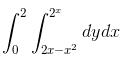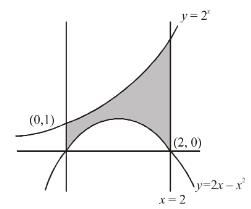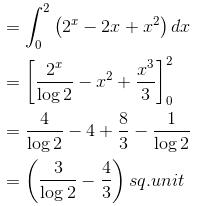The correct answer is: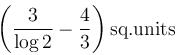QUESTION: 2

### The area of the region inside the curve r = a cosθ and outside the cardioid r = a(1 – cos θ)  will be :

Solution:

To find the intersection, eliminating r between the two equations, i.e.,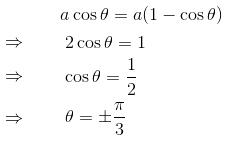∴   The required area will be given by,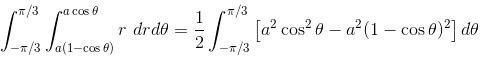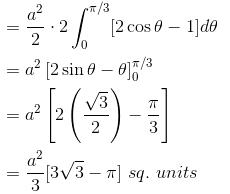The correct answer is: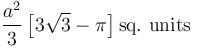QUESTION: 3

### The area bounded by the curves  y2 = x – 1, 2y = x, x – axis and y – axis will be equal to :

Solution:

The required area will be given by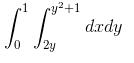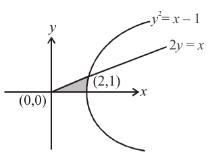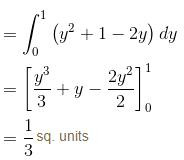The correct answer is: 1/3 sq.units

QUESTION: 4

The area contained between the curve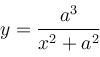and x – axis is :

Solution:

The curve is symmetrical about y-axis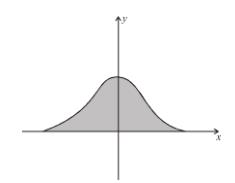Hence, the required area will be,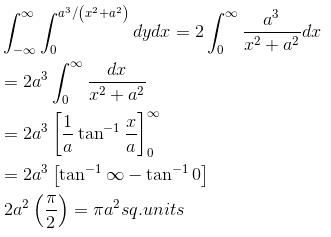The correct answer is: πa2 sq. units

QUESTION: 5

The area included between the circles r = 2acosθ, r = 2bcosθ; a > b  is equal to :

Solution: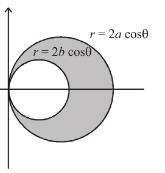The required area will be given by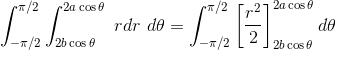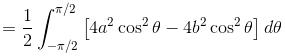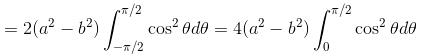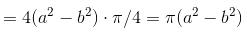The correct answer is: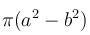QUESTION: 6

The area of the region R, R : r = a(2 – cos2θ),  0 < θ < 2π will be :

Solution:

The required area will be given by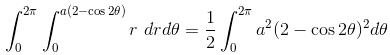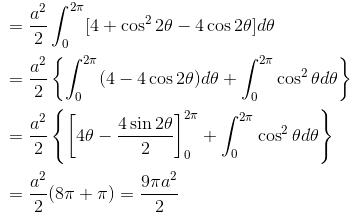The correct answer is: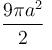QUESTION: 7

The area of the cardioid r = a(1 + cosθ)  lying above x–axis is :

Solution:

The area of the given cardio-id will be given by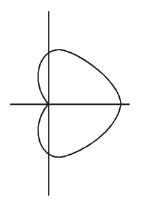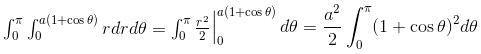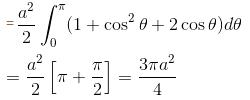The correct answer is: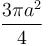QUESTION: 8

The area lying inside the cardioid r = a(1 + cos θ) and outside the circle r = a is :

Solution: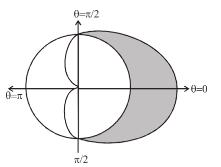The region of integration is enclosed by r = ar = a(1 + cosθ),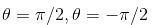∴   The required area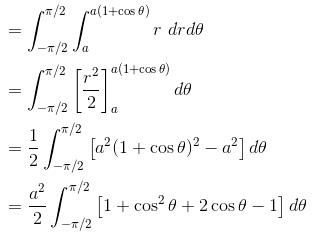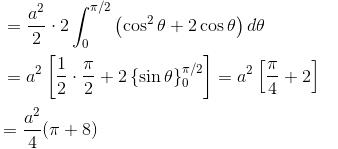The correct answer is: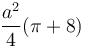QUESTION: 9

The area bounded by the curve y = log x and y = (log x)2 is :

Solution:

To find the point of intersection,
log x = (log x)2
⇒  log x (log x – 1) = 0
⇒  log x = 0        or    log x = 1
⇒  x = 1, e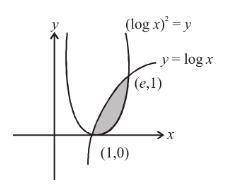Hence, the required area will be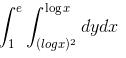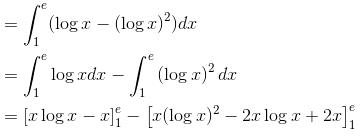= [e - e - (-1)] - [e - 2e + 2e - 2]
= 1 - (e - 2)
= (3 - e) sq. units

The correct answer is: (3 - e) sq.units

QUESTION: 10

The area bounded by y = 1 + 2sin2x, x – axis, x = 0 and  x = π  is :

Solution:

For x ∈ (0, π) y = 1 + sin2x is a non negative, function.
∴ The area bounded. by y = 1 + 2sin2xx-axis, x = 0 and x = π will be in first quadrant.
∴ The area required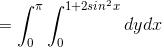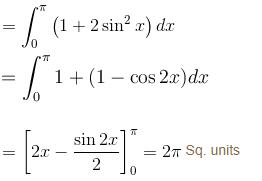The correct answer is: 2π sq. units